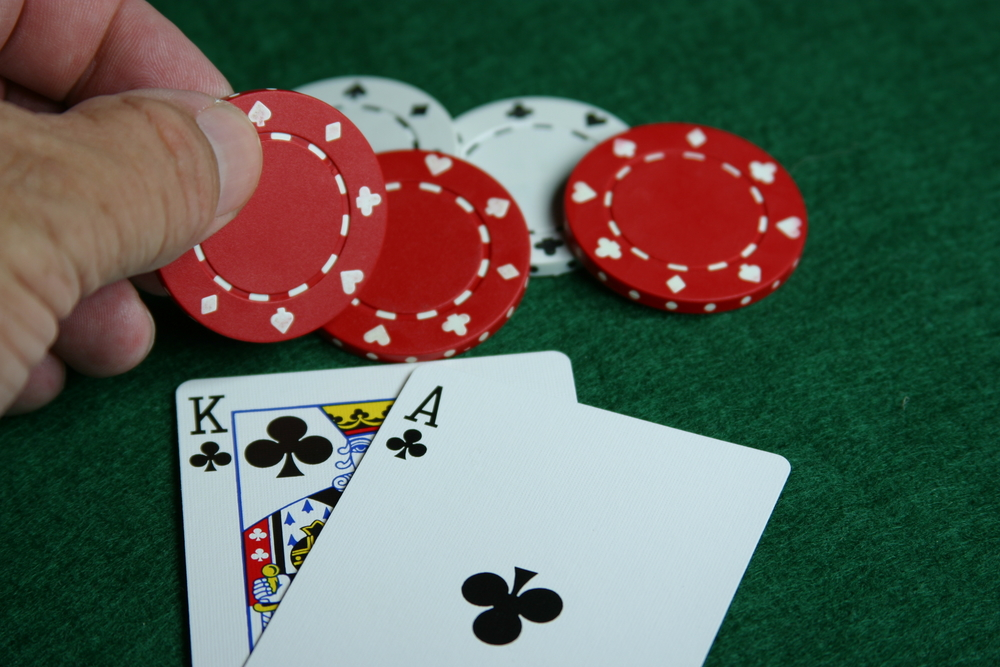# Casino knowledge for the novice – Poker pot odds and equity explainedHave you ever wondered how you can get a handle on a poker game odds, say, Texas hold’em? Well, the one thing that can help you with that is learning how you can count all your odds. Essentially knowledge on poker hand probability use combined with an understanding of the odds used is all that the poker game is all about.

However, doing that means you are only halfway. The next important thing after having the odds is the calculation of your equity in the pot. You will then have to make a comparison between the pot odds and equity to know the correct play in each situation.

So, what are pot odds in poker games?

### Defining what are pot odds

The relationship or connection between pot size and the size of the bet is referred to as pot odds. For instance getting a pot odds of 7 to 1 means that if there is a £ 70 in the pot then you have to call £ 2.

After understanding what pot odds is, you need to know the size of the poker pot odds used.

### Size of a poker pot

Always understand the size of the pot. In the limit, poker doesn’t count the amount of money but rather count the number of bets that are found in the pot. In Texas hold’em if the bets double you need to count the big bets in the pot as two small bets. However, sometimes it is hard to count the pots such as in Pot-Limit or No-Limit. Nevertheless, always count your odds.

### Poker pot odds used

Now you know what you pot odds are, therefore, the next step is utilising them appropriately. The information on pot odds gathered earlier in the article can now be used to make a connection between the value of your hand with the pot odds.

The connection between the two will help you understand the chances you have of making a better hand than your opponents by putting them on likely hands. For instance, if you have a flush draw in Hold’em, say, a four-set of cards that are likely to improve to a flush K♣ 9♣ 8♣ 5♣ and the opponent has a top pair, there are possible 9 cards that will give you flush on flopping four cards to a flush. The 9 cards are also called outs. Also if you check the odds chart and note that it gives a 2:1 chance, then you have will need odds that are not below 2-1 so that you can make a call.

Before you start making these calculations you need to know how to count your outs

Any card that makes your hand is called an out. In the example above there will be 9outs remaining in the card deck for you to get a flush. The 9 outs are as a result of subtraction of the total 13 card suits in a deck and the 4 suits you have.

So with this in mind let’s continue with the drawing odds used by looking into implied odds.

### What is implied odds?

Implied odds is the relationship that exists between current pot size and the expected winning pot. In most cases, the pot will not lay out the correct odds. This is majorly due to the fact that you always expect to win more on hitting your hand.

### Pot odds calculator; is it a necessity?

There are many pot odds calculator that you can find online and use, but you don’t really need them because you have all the necessary tools in your head. Furthermore, you can’t pull out of the poker table and start searching for a pot odd calculator to use.

So what are the odds of you getting that win? This can only be figured out by calculating poker equity. `

### Calculating poker equity

Calculation of equity will help you determine your odds of winning a given pot. Therefore you have to first determine how many outs you have. With practice, you will be good to go. You can use the earlier explanation to make your calculations.

Also, remember the anti-outs idea. For instance a straight complete the opponents flush, the straight cards, therefore, cannot be counted as outs.

### Equity shortcuts

• There are two rules to remember; outs are multiplied by four if you are on the flop and also multiplied by two if you are on the turn.
• An 8 outs means you have a 32% chance of making a straight. More than 8 you can use the formula below
• (number of outs × 4) – (number of outs – 8) = Equity
• For 15 outs in an open-ended straight flush draw, the chance is 53%.
• Combining equity and pot odds
• Memorization of the above formula and techniques and some practice of calculating these odds in your head will prove helpful in the poker table or even in the online poker used to make the calls.
• Implied odds has a big impact on poker game such as No limit Hold’em.